﻿ 大规模无线传感器网络中稀疏信号的数据收集策略 The Data Collection Scheme for Sparse Signals in Large-Scale Wireless Sensor Networks

Computer Science and Application
Vol. 09  No. 03 ( 2019 ), Article ID: 29221 , 14 pages
10.12677/CSA.2019.93063

The Data Collection Scheme for Sparse Signals in Large-Scale Wireless Sensor Networks

Ruiqing Li, Hui Wang

Zhejiang Normal University, Jinhua Zhejiang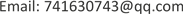Received: Feb. 22nd, 2019; accepted: Mar. 6th, 2019; published: Mar. 13th, 2019ABSTRACT

In a successfully deployed wireless sensor network, the sensing data taken directly from the sensor nodes are subject to a large amount of redundancy due to their temporal and spatial correlations. Therefore, we need to design an effective data collection scheme. Compressive sensing is a popular data collection technology. It includes three core topics: sparse representation of signals, design of observation matrix, and signal reconstruction. This paper focuses on the sparsity of signals. Firstly, the sparsity performance is analyzed from two aspects: real signals and synthetic signals. In compressive sensing, different orthogonal transforms make the signal different levels of sparsity. In order to study the sparsity of the signal further, we have designed two orthogonal transforms, namely Row-trans transform and Col-trans transform. It is found through experiments that the sparse performance of Fourier transform, discrete cosine transform and wavelet transform is relatively poor. The sparse performance of Row-trans transform and the Col-trans transform is better. As far as the reconstruction error is concerned, in the 1800 experimental measurements, the reconstruction errors of the signal under the Row-trans transform and the Col-trans transform are relatively stable, and the error rate are low, which is obviously superior to other orthogonal transforms. The reconstructed signal is closer to the original signal.

Keywords:Wireless Sensor Network, Compressive Sensing, Sparsity, Orthogonal Transforms, Reconstruction Error1. 引言

2. 相关知识

2.1. RIP性质

$\left(1-\delta \right){‖x‖}_{2}^{2}\le {‖\Phi x‖}_{2}^{2}\le \left(1+\delta \right){‖x‖}_{2}^{2}$ (2-1)

${\delta }_{s}=\underset{S\subset \left[N\right],card\left(S\right)\le s}{\mathrm{max}}{‖{\Phi }_{S}^{*}{\Phi }_{S}-Id‖}_{2\to 2}$ (2-2)

RIP性质保证了观测矩阵不会把两个不同的K稀疏信号映射到同一个集合中，即保证原空间到稀疏空间的一一映射关系，它要求从观测矩阵中抽取的每M个列向量构成的矩阵是非奇异的，满足RIP的关键是如何确定非零系数的位置来构造出一个可解的M × K线性方程组。

2.2. 可压缩性数据

${|\theta |}_{\left(1\right)}\ge {|\theta |}_{\left(2\right)}\ge \cdots \ge {|\theta |}_{\left(N\right)}$ ，则第i个变换系数满足

 (2-3)

2.3. 随机投影

${\Phi }_{i,j}=\left\{\begin{array}{l}+1\text{\hspace{0.17em}}\text{\hspace{0.17em}}\text{\hspace{0.17em}}\text{\hspace{0.17em}}prob.1/2\\ -1\text{\hspace{0.17em}}\text{\hspace{0.17em}}\text{\hspace{0.17em}}\text{\hspace{0.17em}}prob.1/2\end{array}$ (2-4)

k个随机投影  可以产生数据x的近似值 $\stackrel{^}{x}$ ，误差为

$‖x-\stackrel{^}{x}‖\le {\beta }_{p}R{\left(k/\mathrm{log}N\right)}^{-1/p+1/2}$

2.4. 稀疏随机投影

${\Phi }_{i,j}=\sqrt{s}\left\{\begin{array}{l}+1\text{\hspace{0.17em}}\text{\hspace{0.17em}}\text{\hspace{0.17em}}\text{\hspace{0.17em}}prob.1/2s\\ 0\text{\hspace{0.17em}}\text{\hspace{0.17em}}\text{\hspace{0.17em}}\text{\hspace{0.17em}}\text{\hspace{0.17em}}\text{\hspace{0.17em}}\text{\hspace{0.17em}}prob.1-1/s\\ -1\text{\hspace{0.17em}}\text{\hspace{0.17em}}\text{\hspace{0.17em}}\text{\hspace{0.17em}}prob.1/2s\end{array}$ (2-5)

3. 压缩感知

3.1. 压缩感知框架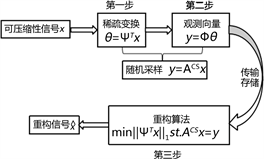Figure 1. Compression sensing framework

3.2. 压缩感知数学模型

3.2.1. 稀疏信号的表示

$\theta ={\left[{\Psi }_{1}^{\text{T}}x,{\Psi }_{2}^{\text{T}}x,\cdots ,{\Psi }_{N}^{\text{T}}x\right]}^{\text{T}}$ (3-1)

3.2.2. 观测向量

$y=\Phi x=\Phi \Psi \theta ={A}^{CS}\theta$ (3-2)

3.2.3. 重构信号

$\stackrel{^}{\theta }=\underset{x\in {R}^{N}}{\mathrm{min}}{‖\theta ‖}_{0}\text{\hspace{0.17em}}\text{\hspace{0.17em}}\text{\hspace{0.17em}}\text{\hspace{0.17em}}\text{\hspace{0.17em}}\text{\hspace{0.17em}}\text{\hspace{0.17em}}\text{\hspace{0.17em}}\text{\hspace{0.17em}}\text{\hspace{0.17em}}\text{s}\text{.t}\text{.}\text{\hspace{0.17em}}\text{\hspace{0.17em}}\text{\hspace{0.17em}}y=\Phi \Psi \theta ={A}^{CS}\theta$ (3-3)

$\stackrel{^}{\theta }=\underset{x\in {R}^{N}}{\mathrm{min}}{‖\theta ‖}_{1}\text{\hspace{0.17em}}\text{\hspace{0.17em}}\text{\hspace{0.17em}}\text{\hspace{0.17em}}\text{\hspace{0.17em}}\text{\hspace{0.17em}}\text{\hspace{0.17em}}\text{\hspace{0.17em}}\text{\hspace{0.17em}}\text{\hspace{0.17em}}\text{\hspace{0.17em}}\text{s}\text{.t}\text{.}\text{\hspace{0.17em}}\text{\hspace{0.17em}}\text{\hspace{0.17em}}y=\Phi \Psi \theta ={A}^{CS}\theta$ (3-4)

$\stackrel{^}{x}=\Psi \stackrel{^}{\theta }$ (3-5)

4. 收集信号与正交变换

4.1. 收集信号

4.1.1. 真实信号

${x}_{T}={\left\{{x}_{{T}_{1}},{x}_{{T}_{2}},\cdots ,{x}_{{T}_{100}}\right\}}^{\text{T}}$ 表示100个温度传感器节点采集到的数据， ${x}_{{T}_{i}},i=1,2,\cdots ,100$ 表示第i个节点采集到的数据。

${x}_{H}={\left\{{x}_{{H}_{1}},{x}_{{H}_{2}},\cdots ,{x}_{{H}_{100}}\right\}}^{\text{T}}$ 表示100个湿度传感器节点采集到的物联网数据， ${x}_{{H}_{i}},i=1,2,\cdots ,100$ 表示第i个节点采集到的数据。

${x}_{A}={\left\{{x}_{{A}_{1}},{x}_{{A}_{2}},\cdots ,{x}_{{A}_{100}}\right\}}^{\text{T}}$ 表示100个空气质量传感器节点采集到的物联网数据， ${x}_{{A}_{i}},i=1,2,\cdots ,100$ 表示第i个节点采集到的数据。

${x}_{P}={\left\{{x}_{{P}_{1}},{x}_{{P}_{2}},\cdots ,{x}_{{P}_{100}}\right\}}^{\text{T}}$ 表示100个光敏传感器节点采集到的物联网数据， ${x}_{{P}_{i}},i=1,2,\cdots ,100$ 表示第i个节点采集到的数据。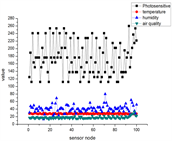(a)(b)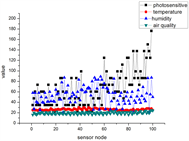(c)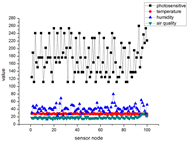(d)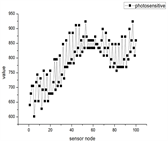(e1)(e2)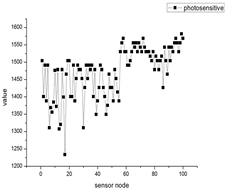(f1)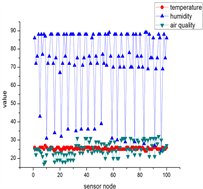(f2)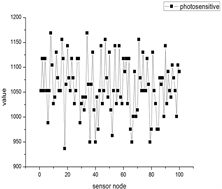(g1)(g2)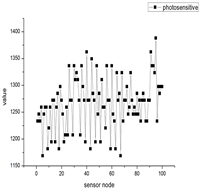(h1)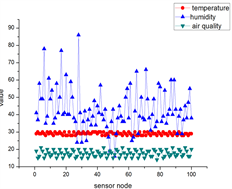(h2)

Figure 2. Sampling signals of different scenes, each sampling number includes 4 kinds of data, namely temperature, humidity, air quality, photosensitive

4.1.2. 人工合成信号

${x}_{1}\left(p,q\right)={x}_{0}+\xi$ (4-1)

$Fm\left(p,q\right)\stackrel{def}{=}\left\{\begin{array}{l}1\text{\hspace{0.17em}}\text{\hspace{0.17em}}\text{\hspace{0.17em}}\text{\hspace{0.17em}}\text{\hspace{0.17em}}\text{\hspace{0.17em}}p+q\le {p}_{low}\text{\hspace{0.17em}}\text{\hspace{0.17em}}\text{\hspace{0.17em}}\text{or}\text{\hspace{0.17em}}\text{\hspace{0.17em}}p+q>{p}_{high}\\ 0\text{\hspace{0.17em}}\text{\hspace{0.17em}}\text{\hspace{0.17em}}\text{\hspace{0.17em}}\text{\hspace{0.17em}}{p}_{low} (4-2)

${x}_{2}\left(p,q\right)={x}_{1}\left(p,q\right)Fm\left(p,q\right)$ (4-3)

4.2. 几种经典的正交变换

4.2.1. 离散傅里叶变换

$X\left[k\right]=\underset{n=0}{\overset{N-1}{\sum }}x\left(n\right){\text{e}}^{-i\frac{\text{2π}}{N}nk}=\underset{n=0}{\overset{N-1}{\sum }}x\left(n\right){W}_{N}^{nk},\text{\hspace{0.17em}}\text{\hspace{0.17em}}\text{\hspace{0.17em}}n,k=0,1,\cdots ,N-1,\text{\hspace{0.17em}}{W}_{N}={\text{e}}^{-i\frac{\text{2π}}{N}}$ (4-4)

$\left[\begin{array}{c}X\left(0\right)\\ X\left(1\right)\\ X\left(2\right)\\ ⋮\\ X\left(N-1\right)\end{array}\right]=\left[\begin{array}{ccccc}1& 1& 1& \cdots & 1\\ 1& {W}_{N}^{1}& {W}_{N}^{2}& \cdots & {W}_{N}^{\left(N-1\right)}\\ 1& {W}_{N}^{2}& {W}_{N}^{4}& \cdots & {W}_{N}^{2\left(N-1\right)}\\ ⋮& ⋮& ⋮& \ddots & ⋮\\ 1& {W}_{N}^{\left(N-1\right)}& {W}_{N}^{2\left(N-1\right)}& \cdots & {W}_{N}^{\left(N-1\right)\left(N-1\right)}\end{array}\right]\left[\begin{array}{c}x\left(0\right)\\ x\left(1\right)\\ x\left(2\right)\\ ⋮\\ x\left(N-1\right)\end{array}\right]$

$X={W}_{N}x$ ，其中 ${W}_{N}$ 为变换矩阵，将变换矩阵 ${W}_{N}$ 乘以一个系数 $1/\sqrt{N}$ 使其变为正交矩阵可得离散傅里叶变换基(矩阵)：

${\stackrel{˜}{W}}_{N}=\frac{1}{\sqrt{N}}\left[\begin{array}{ccccc}1& 1& 1& \cdots & 1\\ 1& {W}_{N}^{1}& {W}_{N}^{2}& \cdots & {W}_{N}^{\left(N-1\right)}\\ 1& {W}_{N}^{2}& {W}_{N}^{4}& \cdots & {W}_{N}^{2\left(N-1\right)}\\ ⋮& ⋮& ⋮& \ddots & ⋮\\ 1& {W}_{N}^{\left(N-1\right)}& {W}_{N}^{2\left(N-1\right)}& \cdots & {W}_{N}^{\left(N-1\right)\left(N-1\right)}\end{array}\right]$ (4-5)

4.2.2. 离散余弦变换

${f}_{m}=\frac{1}{2}\left({x}_{0}+{\left(-1\right)}^{m}{x}_{N-1}\right)+\underset{k=1}{\overset{N-2}{\sum }}{x}_{k}\mathrm{cos}\left[\frac{\text{π}}{N-1}mk\right],\text{\hspace{0.17em}}\text{\hspace{0.17em}}\text{\hspace{0.17em}}\text{\hspace{0.17em}}\text{\hspace{0.17em}}\text{\hspace{0.17em}}\text{\hspace{0.17em}}\text{\hspace{0.17em}}k=0,1,\cdots ,N$ (4-6)

${\stackrel{˜}{C}}_{N}=\frac{1}{\sqrt{N}}\left[\begin{array}{cccc}1& 1& \cdots & 1\\ \sqrt{2}\mathrm{cos}\left(\frac{\text{π}}{2N}\right)& \sqrt{2}\mathrm{cos}\left(\frac{3\text{π}}{2N}\right)& \cdots & \sqrt{2}\mathrm{cos}\left(\frac{\left(2N-1\right)\text{π}}{2N}\right)\\ ⋮& ⋮& \ddots & ⋮\\ \sqrt{2}\mathrm{cos}\left(\frac{\left(N-1\right)\text{π}}{2N}\right)& \sqrt{2}\mathrm{cos}\left(\frac{3\left(N-1\right)\text{π}}{2N}\right)& \cdots & \sqrt{2}\mathrm{cos}\left(\frac{\left(N-1\right)\left(2N-1\right)\text{π}}{2N}\right)\end{array}\right]$ (4-7)

4.2.3. 小波变换

$f\left(t\right)$ 是平方可积函数 $f\left(t\right)\in {L}^{2}\left(R\right)$$\psi \left(t\right)$ 是基本小波或母小波函数，则小波变换公式如下：

$WT\left(a,\tau \right)=\frac{1}{\sqrt{a}}{\int }_{-\infty }^{\infty }f\left(t\right)\psi \left(\frac{t-\tau }{a}\right)\text{d}t$ (4-8)

$\left\{{\psi }_{j,k}\left(t\right)={2}^{j/2}\psi \left({2}^{j}t-k\right)|j,k\in Z\right\}$

 (4-9)

$\psi \left(t\right)=\left\{\begin{array}{l}1\text{\hspace{0.17em}}\text{\hspace{0.17em}}\text{\hspace{0.17em}}\text{\hspace{0.17em}}\text{\hspace{0.17em}}\text{\hspace{0.17em}}\text{\hspace{0.17em}}\text{\hspace{0.17em}}\text{\hspace{0.17em}}\text{\hspace{0.17em}}0\le t\le 1\\ 0\text{\hspace{0.17em}}\text{\hspace{0.17em}}\text{\hspace{0.17em}}\text{\hspace{0.17em}}\text{\hspace{0.17em}}\text{\hspace{0.17em}}\text{\hspace{0.17em}}\text{\hspace{0.17em}}\text{\hspace{0.17em}}\text{otherwise}\end{array}$ (4-10)

4.3. 设计正交变换

4.3.1. Row-trans变换(4-11)

${\psi }_{2}=\left[\begin{array}{cccc}{x}_{1}& {x}_{2}& \cdots & {x}_{K}\\ |{x}_{2K}-{x}_{1}|& |{x}_{2K-1}-{x}_{2K-2}|& \cdots & |{x}_{K+1}-{x}_{K}|\\ |{x}_{2K+1}-{x}_{2k}|& |{x}_{2K+2}-{x}_{2K-1}|& \cdots & |{x}_{3K}-{x}_{K+1}|\\ ⋮& ⋮& & ⋮\\ |{x}_{\left(K-1\right)K}-{x}_{\left(K-3\right)K}|& |{x}_{\left(K-1\right)K-1}-{x}_{\left(K-3\right)K+1}|& \cdots & |{x}_{\left(K-2\right)K+1}-{x}_{\left(K-2\right)K}|\\ |{x}_{\left(K-1\right)K+1}-{x}_{\left(K-1\right)K}|& |{x}_{\left(K-1\right)K+2}-{x}_{\left(K-1\right)K-1}|& \cdots & |{x}_{N}-{x}_{N-2K}|\end{array}\right]$ (4-12)

${\psi }_{3}\left(i,j\right)={\psi }_{2}\left(i,j\right)×\frac{1}{{\psi }_{2}\left(i,j\right)}$ (4-13)

 (4-14)

$\begin{array}{c}{\psi }^{\text{T}}\psi ={\left[\begin{array}{cc}{\psi }_{4}& \\ & I\end{array}\right]}^{\text{T}}\left[\begin{array}{cc}{\psi }_{4}& \\ & I\end{array}\right]\\ =\left[\begin{array}{cc}{\psi }_{4}^{\text{T}}{\psi }_{4}& \\ & {I}^{\text{T}}I\end{array}\right]\\ =E\end{array}$

4.3.2. Col-trans变换

${\psi }_{1}=\left[\begin{array}{cccc}{x}_{1}& {x}_{2}& \cdots & {x}_{K}\\ {x}_{2K}& {x}_{2K-1}& \cdots & {x}_{K+1}\\ {x}_{2K+1}& {x}_{2K+2}& \cdots & {x}_{3K}\\ ⋮& ⋮& & ⋮\\ {x}_{\left(K-1\right)K}& {x}_{\left(K-1\right)K-1}& \cdots & {x}_{\left(K-2\right)K}\\ {x}_{\left(K-1\right)K+1}& {x}_{\left(K-1\right)K+2}& \cdots & {x}_{N}\end{array}\right]$ (4-15)

${\psi }_{2}=\left[\begin{array}{cccc}{x}_{1}& |{x}_{2}-{x}_{1}|& \cdots & |{x}_{K}-{x}_{K-1}|\\ {x}_{2K}& |{x}_{2K-1}-{x}_{2K}|& \cdots & |{x}_{K+1}-{x}_{K+2}|\\ {x}_{2K+1}& |{x}_{2K+2}-{x}_{2K+1}|& \cdots & |{x}_{3K}-{x}_{3K-1}|\\ ⋮& ⋮& & ⋮\\ {x}_{\left(K-1\right)K}& |{x}_{\left(K-1\right)K-1}-{x}_{\left(K-1\right)K}|& \cdots & |{x}_{\left(K-2\right)K+1}-{x}_{\left(K-2\right)K+2}|\\ {x}_{\left(K-1\right)K+1}& |{x}_{\left(K-1\right)K+2}-{x}_{\left(K-1\right)K+1}|& \cdots & |{x}_{N}-{x}_{N-1}|\end{array}\right]$ (4-16)

${\psi }_{3}\left(i,j\right)={\psi }_{2}\left(i,j\right)×\frac{1}{{\psi }_{2}\left(i,j\right)}$ (4-17)

 (4-18)

5. 实验

$\epsilon =\frac{{‖x-\stackrel{^}{x}‖}_{2}}{{‖\stackrel{^}{x}‖}_{2}}$ (5-1)

5.1. 搭建实验平台

5.2. 实验结果及分析

1) 信号在不同正交变换下的稀疏度的表示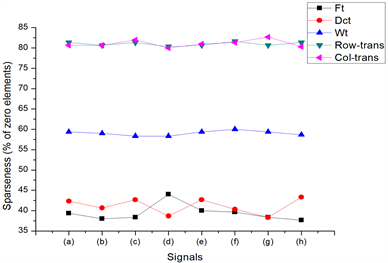Figure 3. The sparsity of sampling signals of different scene under different orthogonal transformations

2) 真实信号在不同正交变换下的重构误差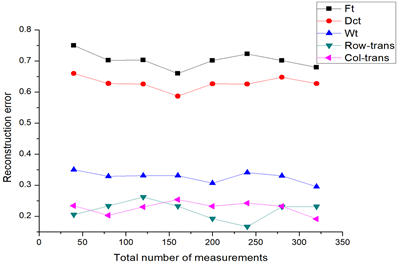Figure 4. Reconstruction error of sampled signals under different orthogonal transforms

3) 合成信号在不同正交变换下的重构误差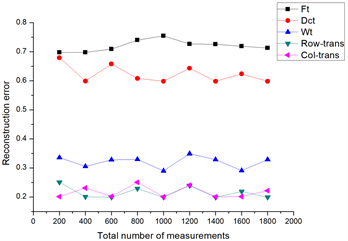Figure 5. Reconstruction error of synthesized signals under different orthogonal transforms

6. 结论及未来的工作

The Data Collection Scheme for Sparse Signals in Large-Scale Wireless Sensor Networks[J]. 计算机科学与应用, 2019, 09(03): 551-564. https://doi.org/10.12677/CSA.2019.93063

1. 1. Gupta, P. and Kumar, P.R. (2000) The Capacity of Wireless Networks. IEEE Transactions on Information Theory, 46, 388-404. https://doi.org/10.1109/18.825799

2. 2. Birkhauser, A. (2010) Mathematical Introduction to Compressive Sensing. University of Maryland, College Park, MD, USA, 1-33.

3. 3. Bajwa, W., Haupt, J., Sayeed, A. and Nowak, R. (2006) Compressive Wireless Sens-ing. Proceedings of the International Conference on Information Processing in Sensor Networks (IPSN), Nashville, 19-21 April 2006, 134-142.

4. 4. Baron, D., Duarte, M.F., Sarvotham, S., Wakin, M.B. and Baraniuk, R. (2005) An Information-Theoretic Approach to Distributed Compressed Sensing. Proceedings of the 43rd Allerton Conference on Communication, Control, and Computing, 28-30 September 2005.

5. 5. Candès, E.J. and Romberg, J. (2006) Robust Uncertainty Principles: Exact Signal Reconstruction from Highly Incomplete Frequency Information. IEEE Transactions on Information Theory, 52, 489-508. https://doi.org/10.1109/TIT.2005.862083

6. 6. Candes, E.J. and Tao, T. (2006) Near-Optimal Signal Recovery from Random Projections: Universal Encoding Strategies? IEEE Transactions on Information Theory, 52, 5406-5424. https://doi.org/10.1109/TIT.2006.885507

7. 7. Luo, C., Wu, F., Sun, J. and Chen, C.W. (2009) Compressive Data Gathering for Large-Scale Wireless Sensor Networks. Proceedings of ACM Mobicom’09, Beijing, 20-25 September 2009, 145-155. https://doi.org/10.1145/1614320.1614337

8. 8. Elad, M. (2010) Sparse and Redundant Representations. The Technion-Israel In-stitute of Technology Haifa, Israel, 8-12. https://doi.org/10.1007/978-1-4419-7011-4

9. 9. Quert, G., Masierot, R., Munaretto, D., Rossit, M., Widmer, J. and Zorzit, M. (2009) On the Interplay between Routing and Signal Representation for Compressive Sensing in Wireless Sensor Networks. 2009 Information Theory & Applications Workshop, San Diego, 8-13 February 2009, 1-10.

10. 10. Liu, X., Luo, J. and Vasilakos, A.V. (2011) Compressed Data Aggregation for Energy Efficient Wireless Sensor Networks. 2011 8th Annual IEEE Communications Society Conference on Sensor, Mesh and Ad Hoc Communications and Networks, Salt Lake City, 27-30 June 2011, 46-54.

11. 11. Guo, L., Beyah, R. and Li, Y. (2011) SMITE: A Stochastic Compressive Data Collection Protocol for Mobile Wire-less Sensor Networks. 2011 Proceedings IEEE INFOCOM, Shanghai, 10-15 April 2011, 1611-1619. https://doi.org/10.1109/INFCOM.2011.5934953

12. 12. Zheng, H.F., Yang, F., Tian, X.H., Gan, X.Y. and Xiao, S.L. (2015) Data Gathering with Compressive Sensing in Wireless Sensor Networks: A Random Walk Based Approach. IEEE Transactions on Parallel and Distributed Systems, 26, 35-45. https://doi.org/10.1109/TPDS.2014.2308212

13. 13. Wang, W., Garofalakis, M. and Ram-chandran, K. (2007) Distributed Sparse Random Projections for Refinable Approximation. 2007 6th International Symposium on In-formation Processing in Sensor Networks, Cambridge, 25-27 April 2007, 331-339. https://doi.org/10.1109/IPSN.2007.4379693

14. 14. Liu, X.-Y., Zhu, Y.M., Kong, L.H., Liu, C., Gu, Y., Vasilakos, A.V. and Wu, M.-Y. (2015) CDC: Compressive Data Collection for Wireless Sensor Networks. IEEE Transactions on Parallel and Distributed Sys-tems, 26, 2188-2196. https://doi.org/10.1109/TPDS.2014.2345257

15. 15. Ebrahimi, D. and Assi, C. (2014) Compressive Data Gathering Using Random Projection for Energy Efficient Wireless Sensor Networks. Ad Hoc Networks, 16, 105-119. https://doi.org/10.1016/j.adhoc.2013.12.004

16. 16. Baron, D., Sarvotham, S. and Baraniuk, R.G. (2010) Bayesian Compressive Sensing via Belief Propagation. IEEE Transactions on Signal Processing, 58, 269-280. https://doi.org/10.1109/TSP.2009.2027773

17. 17. Li, P., Hastie, T.J. and Church, K.W. (2006) Very Sparse Random Projections. Proceedings of the ACM International Conference on Knowledge Discovery and Data Mining, Philadelphia, 20-23 August 2006, 287-296. https://doi.org/10.1145/1150402.1150436

18. 18. Haupt, J., Bajwa, W.U., Rabbat, M. and Nowak, R. (2008) Compressed Sensing for Networked Data. IIEEE Signal Processing Magazine, 25, 92-101. https://doi.org/10.1109/MSP.2007.914732

19. 19. Strang, G. (2003) Introduction to Linear Algebra. Third Edition, Massachusetts Institute of Technology, 184-201, 352-359.

20. 20. Anna, N. and NoraDanil, M. (2004) Convex Optimization. Cambridge University Press, Cambridge, 1-8.